# 4.1 Electrostatic Potential and the POISSON Equation

Planar CNT-FETs constitute the majority of devices fabricated to date, mostly due to their relative simplicity and moderate compatibility with existing manufacturing technologies. However, coaxial devices (see Fig. 4.1) are of special interest because their geometry allows for better electrostatic control than their planar counterparts. These devices would exhibit wrap-around gates that maximize capacitive coupling between the gate electrode and the CNT channel. Presently, the closest approximation to this geometry are electrolyte-gated devices [232,233]. Alternative structures that place CNTs vertically with respect to the substrate have already been used for field-emission applications [234,235]. Coaxial CNT-FETs could be fashioned by placing CNTs inside the cavities of a porous material such as alumina, surrounding them by gate electrodes.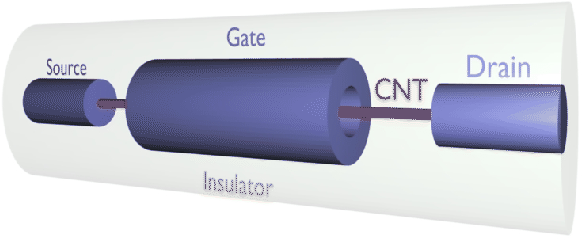Appropriate treatment of the electrostatic potential in the device is essential for accurately predicting the device characteristics . The electron-electron interaction self-energy of lowest order yields the HARTREE potential, which is the solution of the POISSON equation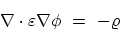(4.1)

where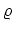is the total charge density, given (in cylindrical coordinates) by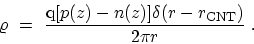(4.2)

Here,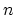and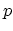denote the electron and hole concentration per unit length, respectively, and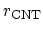is the radius of the CNT. In (4.2)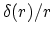is the DIRAC delta function in cylindrical coordinates, implying that the carrier density is approximated by a sheet charge distributed along the insulator-CNT interface . Due to azimuthal symmetry in wrap-around gate devices, the carriers are uniformly distributed as a function of the azimuthal angle.
Subsections M. Pourfath: Numerical Study of Quantum Transport in Carbon Nanotube-Based Transistors